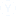what is shortcut for solving ratio and proportion
Solving ratio and proportion problems using shortcuts
• GS
• Gokul
• 08 अक्तूबर
• 836 दृश्य
• 1 उत्तर1 उत्तर
1-1 of  1
• first of all u have to read the concept of Ratio and proportion and keep them fixed in ur mind...........
what is Ratio ? The number of times one quantity contains another quantity of the same kind is called the ratioof the two quantities..
now its type-
Consequent >
In the ratio 2 :3 , 2 is the antecedent and 3 is the consequent.
Compound Ratio > Ratios are compound by multiplying together the antecedents for a new antecedent, and the consequents for a new consequent.
Inverse Ratio > If 2:3 be the given ratio, then 1/2: 1/3  or 3 :2 is called its inverse or reciprocal ratio.
Now what is Proportion ?
Consider the two ratios:

1st ratio                      2nd ratio
6 : 18                           8 : 24
Since 6 is one-third of 18, and 8 is one –third of 24, the two ratios are equal. The equality of ratio is called proportion.
The number 6, 18, 8 and 24 are said to be in proportion.अन्य संबंधित चर्चाएंमॉक परीक्षण अभ्यास के लिए
IBPS PO Prelim Exam